# C++ Program to Count the Occurrence of a Digit in a Number

The C++ program to count the occurrence of a digit in a number is a simple program that receives two inputs – a number and a digit

It counts the number of times a digit appears in the given number and prints the output. The occurrence is 0 when the digit is not in the number.

This program is intended for beginners of C++ programming language. We used Dev-C++ compiler – version 4.9.9.2 installed on a Windows 7 64-bit computer. This program code will work with any standard C++ compiler if the correct header files are used.

You will find the following section to help you understand the program logic.

• Problem Definition
• Flowchart of the program.
• Program Source Code
• Verified Output after running the program.

## Problem Definition

The program need two inputs as follows

1. A positive integer number
2. A positive digit

If you do not use positive value the program fails, so make sure that the inputs are always positive number.

We use 4 steps to solve the problem of counting the occurrence of a digit. The occurrence is stored in a variable called count.

1. When the number is given, each digit must be separated.
2. Extracted digit is compared with the input digit.
3. If there is a match – (digit == extracted digit), then count is incremented by 1, otherwise check the next extracted digit, until all the digits in the given number is exhausted.
4. Print the counted value as output.

## Flowchart – Count the Occurrence of a Digit

A flowchart of the program show that we make two comparisons during the execution of the program.

1. Check if all the digits in the given number is checked – (while (quotient! = 0)).
2. Check if extracted digits from the number is equal to input digit so that we can count the occurrence – if (digit == remainder).

## Program Code – Count the Occurrence of a Digit

/* C++ Program to Count the Occurrence of a Digit in a Number */
#include <cstdlib>
#include <iostream>
#include <conio.h>

using namespace std;
int main()
{
int number, remainder, quotient;
int count, digit, i;
count = digit = i = 0;

cout << "\n\n\n\n";
cout << "\t\t\tEnter the number:";
cin >> number;
cout << "\t\t\tEnter the digit:";
cin >> digit;
quotient = number;

while (quotient! = 0)
{
remainder = quotient % 10;
quotient = quotient / 10;
if (digit == remainder)
{
count = count + 1;
}
}
cout << "\n\n\n\n";
cout << "\t\t\tThe digit" << " " << digit << " " << "appeared" << " " << count << " " << "times\n\n";
getch();
return EXIT_SUCCESS;
}

We use two important logic in this program to count the occurrence of a digit.

Remainder = quotient % 10;

The above statement will store the remainder which is extracted digit we want.

For example,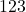will give a remainder of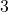.

Quotient = quotient / 10;

The above statement will give next number from which we want to extract a digit.

For example,will extract the digit, but next time we want to extract digit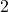which is only possible if we have number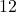.

So, if we divideby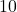, the quotient iswhich is the number we want for next iteration to extract another digit.

## Output

The output of the program is given below.

                                Enter the number:36666
Enter the digit:6

The digit 6 appeared 4 times